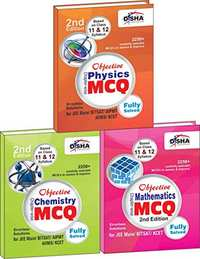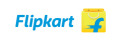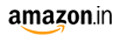NEET (UG) (entrance exam)
Share

# Objective Physics, Chemistry, Mathematics: Chapter-wise MCQ for JEE Main/BITSAT/KCET (Box Set) NEET (UG)M.R.P 770/-450.00(42%)578.00(25%)
Product Description

Objective Physics, Chemistry, Mathematics - Chapter-wise MCQ for JEE Main/BITSAT /KCET is a collection of carefully selected MCQ's for Engineering entrance exams. The book is useful for JEE Main, BITSAT and KCET. The book has been designed on the pattern of class 11 and 12 syllabus as prescribed by NCERT. The book contains ‘Chapter-wise MCQs’ which covers all the important concepts and applications required to crack the mentioned exams. The solutions to each and every question is provided immediately after the questions. The solutions have been prepared in a manner that a student can easily understand them. The book contains 29/30/30 chapters covering a total of 9000+ MCQs, in Physics,Chemistry and Maths respectively, with solutions. This is an ideal book to practice and revise the complete syllabus of the mentioned exams.

Book 1 - Physics

• Physical World, Units and Measurements
• Motion in a Straight Line
• Motion in a Plane
• Laws of Motion
• Work, Energy and Power
• System of Particles and Rotational Motion
• Gravitation
• Mechanical Properties of Solids
• Mechanical Properties of Fluids
• Thermal Properties of Matter
• Thermodynamics
• Kinetic Theory
• Oscillations
• Waves
• Electric charges and Fields
• Electrostatic Potential and Capacitance
• Current Electricity
• Moving Charges and Magnetism
• Magnetism and Matter
• Electromagnetic Induction
• Alternating Current
• Electromagnetic Waves
• Ray Optics and Optical Instruments
• Wave Optics
• Dual Nature of Radiation and Matter
• Atoms
• Nuclei
• Semiconductor Electronics : Material, Devices and Simple Circuits
• Communication Systems

Book 2 - Chemistry

• Some Basic Concepts of Chemistry
• Structure of Atom
• Classification of Elements and Periodicity in Properties
• Chemical Bonding and Molecular Structure
• States of Matter
• Thermodynamics
• Equilibrium
• Redox Reactions
• Hydrogen
• The s-Block Elements
• The p-Block Elements (Group 13 and 14)
• Organic Chemistry - Some Basic Principles and Techniques
• Hydrocarbons
• Environmental Chemistry
• The Solid State
• Solutions
• Electrochemistry
• Chemical Kinetics
• Surface Chemistry
• General Principles and Processes of Isolation of Elements
• The p-Block Elements (Group 15, 16, 17 and 18)
• The d and f-Block Elements
• Coordination Compounds
• Haloalkanes and Haloarenes
• Alcohols, Phenols and Ethers
• Aldehydes, Ketones and Carboxylic acids
• Amines
• Biomolecules
• Polymers
• Chemistry in Everyday Life

Book 3 - Mathematics

• Sets
• Relations and Functions I
• Trigonometric Functions
• Principle of Mathematical Induction
• Complex Numbers and Quadratic Equations
• Linear Inequalities
• Permutations and Combinations
• Binomial Theorem
• Sequences and Series
• Straight Lines
• Conic Sections
• Introduction to Three-Dimensional Geometry
• Limits and Derivatives
• Mathematical Reasoning
• Statistics
• Probability I
• Relations and Functions II
• Inverse Trigonometric Functions
• Matrices
• Determinants
• Continuity and Differentiability
• Application of Derivatives
• Integrals
• Application of Integrals
• Differential Equations
• Vector-Algebra
• Three-Dimensional Geometry
• Linear Programming
• Probability II
• Solution of Triangles and Heights and Distance

S